# Difference between revisions of "Examples in mathematics"

Examples in mathematics have different flavor than examples in other disciplines. This is probably because definitions in mathematics are different from definitions in other disciplines (mathematical definitions are exact). Some common problems of deciding whether something is or is not an example do not appear in mathematics. Instead, there are other problems.

## Unit testing and examples

A common problem in math is that one comes in with some preconceived idea of what an object should "look like" which is different from what the definition says. In other words, there is a mismatch between one's intuitive notion and the definition.

Take the example of a definition of function. A function is some object that takes each object in some set to a unique object in another set. Someone who was not familiar with the formal definition might mistakenly think of a function as "something that is defined by a formula".

Is an example according to definition Is not an example according to definition
Is an example according to intuition An "obvious" example, or central example. Let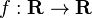$f : \mathbf R \to \mathbf R$ be defined by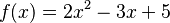$f(x) = 2x^2 - 3x + 5$. This does define a function, and someone who thought that a function is "something that is defined by a formula" would think that this is a function. A surprising non-example.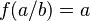$f(a/b) = a$ (i.e. a function that outputs the numerator of a fraction); a function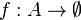$f : A \to \emptyset$ where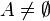$A \ne \emptyset$.
Is not an example according to intuition A surprising example. Let$f : \mathbf N \to \mathbf N$ be defined by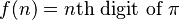$f(n) = n\text{th digit of }\pi$. This does define a function, but someone who thought that a function is "something that is defined by a formula" wouldn't think it is a function.
Another example is the empty function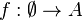$f : \emptyset \to A$ for any set$A$. This does define a function, but the function doesn't "do" anything. Since it is an "extreme" example of a function, someone who was only used to dealing with "normal-looking" functions (or someone who isn't used to working with the empty set or vacuous conditions) might dismiss this example.
As a third example, let$\mathcal M$ be the set of all Turing machines, and let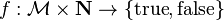$f : \mathcal M \times \mathbf N \to \{\text{true}, \text{false}\}$ be defined by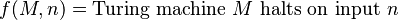$f(M,n) = \text{Turing machine }M\text{ halts on input }n$.
An obvious non-example.

## Hierarchical nature of examples

Something can be considered "concrete" or "abstract" depending on the context. Consider a term like "metric space". One can give examples of metric spaces. On the other hand, a metric space is itself an example (of a structured space, of a topological space).